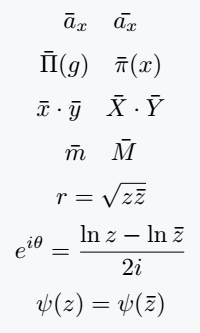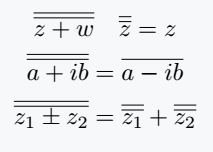# How do you use bar and overline over characters?

Although both overline and bar symbols look similar, there are structural and functional differences between them. For example, bar is only valid for single characters.

But, if there is more than one character, it is best practice to use overline instead of bar.

## Use bar command for a single character

There is a \bar command to represent the bar symbol above a character. But, that single character must be passed as an argument.

So, look at the output below, where the use of not responsive is shown.

\documentclass{article}
\begin{document}
$\bar{a}_x\quad \bar{a_x}$
$\bar{\Pi}(g)\quad \bar{\pi}(x)$
$\bar{x}\cdot\bar{y} \quad \bar{X}\cdot\bar{Y}$
$\bar{m} \quad \bar{M}$
$r=\sqrt{z\bar{z}}$
$e^{i\theta}=\frac{\ln z-\ln \bar{z}}{2i}$
$\psi(z)=\psi(\bar{z})$
\end{document}

Output :As you can see from the output, hopefully, the bar symbol is positioned inconsistently with most of the single character sizes.

It is not correct to use this command for single capital letters.

## Use \overline command for multiple characters

Same process for Overline, just replace the previous command with \overline.

\documentclass{article}
\begin{document}
$\overline{a}_1 ,\overline{a}_2 ,\dots ,\overline{a}_n$
$\overline{m}_1,\overline{m}_2 ,\dots ,\overline{m}_n$
$\overline{z+w}=\overline{z}+\overline{w}$
$\overline{z-w}=\overline{z}-\overline{w}$
$\overline{zw} = \overline{z}\;\overline{w}$
$\overline{\frac{z}{w}}=\frac{\overline{z}}{\overline{w}}$
$\overline{pqr}\quad\overline{overline}$
\end{document}

Output :Logically, for a double bar use the same command twice.

\documentclass{article}
\begin{document}
$\overline{\overline{z+w}}\quad \overline{\overline{z}}=z$
$\overline{\overline{a+ib}}=\overline{a-ib}$
$\overline{\overline{z_1\pm z_2}}=\overline{\overline{z_1}}+\overline{\overline{z_2}}$
\end{document}

Output :#### Md Jidan Mondal

LaTeX expert with over 10 years of experience in document preparation and typesetting. Specializes in creating professional documents, reports, and presentations using LaTeX.